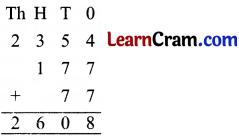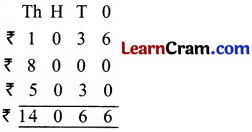# DAV Class 3 Maths Chapter 2 Worksheet 5 Solutions

The DAV Class 3 Maths Book Solutions and DAV Class 3 Maths Chapter 2 Worksheet 5 Solutions of Addition offer comprehensive answers to textbook questions.

## DAV Class 3 Maths Ch 2 WS 5 Solutions

Question 1.
There are 2000 boys and 2520 girls in a school. How many students are there in all?
Solution:
No. of boys = 2000
No. of girls = 2520Total students = 4520
Total students are 4520.

Question 2.
A school planted 1999 trees in one year and 2770 trees in the next year. How many trees were planted in two years?
Solution:
Trees planted in first year = 1999
Trees planted in next year = 2770Total trees planted = 4769
4769 trees were planted in two years.Question 3.
There are 2958 men, 2596 women and 3102 children in a town. What is the total population of the town?
Solution:
No. of men = 2958
No. of women = 2596
No. of children = 3102Total population = 8656
Total population is 8656.

Question 4.
In a library, there are 2585 English story books, 5525 Hindi story books and 1235 Comics. How many books are there in all?
Solution:
No. of English story books = 2585
No. of Hindi story books = 5525
No. of Comics = 1235Total books = 9345
Total books are 9345.### DAV Class 3 Maths Chapter 2 Value Based Questions

Vasanti didi comes …………….. in the school.

Question 1.
How much money did Rahul’s parents and grandmother give to Vasanti?
Solution:
Money given by Rahul’s parents = ₹ 3460
Money given by grandmother = ₹ 1500
= ₹ 3460 + ₹ 15000 = ₹ 4960
Total money = ₹ 4960

Question 2.
How much money did Rahul’s family give to help Vasanti?
Solution:
Rahul’s parents and grandmother gave money = ₹ 4960
Rahul and his sister contributed = ₹ 170
= 4960 + 170 = 5130
Total money given by family = ₹ 5130

Question 3.
Suggest any two ways in which you can help someone like Naval?
Solution:
By giving study materials like note books, chart etc.
(ii) By giving money for shoes.### DAV Class 3 Maths Chapter 2 Worksheet 5 Notes

Word Problems:

Example 1.
Rakhi purchases 2354 red marbles, 177 green marble and 77 white marbles in one day. Find total number of marbles purchased.
Solution:
No. of red marbles = 2354
No. of green marbles = 177
No. of white marbles = 77
Total no. of marbles = 2608Rakhi purchased 2608 marbles.Example 2.
Mr. Shah sold chair for ₹ 1036, sofa for ₹ 8000 and table for ₹ 5030. How much many he get?
Solution:
Chair sold = ₹ 1036
Sofa sold = ₹ 8000
Table sold = ₹ 5030Total money he get = ₹ 14066
Mrs. Shah get ₹ 14066.